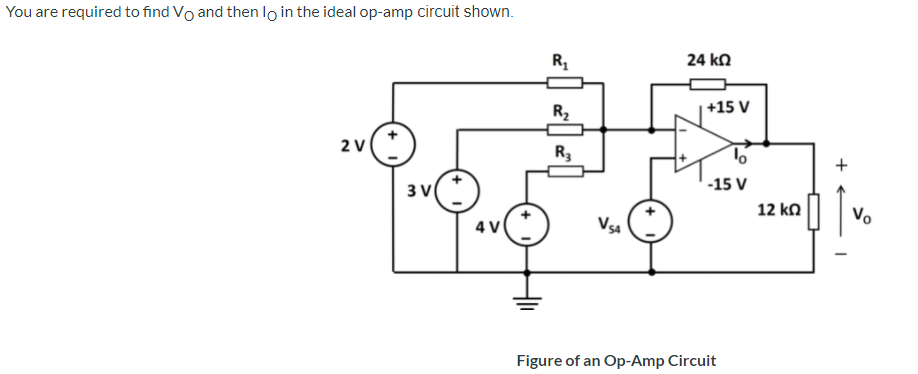# Question Solved1 AnswerR1 = 9 kΩ R2 = 8 kΩ R3 = 5 kΩ VS4 = 4 V 1.) Calculate VO and then IO 2) What is the maximum voltage that VS4 may be increased to before the op-amp goes into positive saturation? You are required to find Vo and then lo in the ideal op-amp circuit shown. R 24 ΚΩ R2 +15 V 2 V R3 + 60 -15 v 12 ΚΩ 3 V 4V V. Vse 1 1 Figure of an Op-Amp Circuit36SRTX The Asker · Electrical Engineering

R1 = 9 kΩ

R2 = 8 kΩ

R3 = 5 kΩ

VS4 = 4 V1.) Calculate VO and then IO

2) What is the maximum voltage that VS4 may be increased to before the op-amp goes into positive saturation?

Transcribed Image Text: You are required to find Vo and then lo in the ideal op-amp circuit shown. R 24 ΚΩ R2 +15 V 2 V R3 + 60 -15 v 12 ΚΩ 3 V 4V V. Vse 1 1 Figure of an Op-Amp Circuit
More
Transcribed Image Text: You are required to find Vo and then lo in the ideal op-amp circuit shown. R 24 ΚΩ R2 +15 V 2 V R3 + 60 -15 v 12 ΚΩ 3 V 4V V. Vse 1 1 Figure of an Op-Amp Circuit